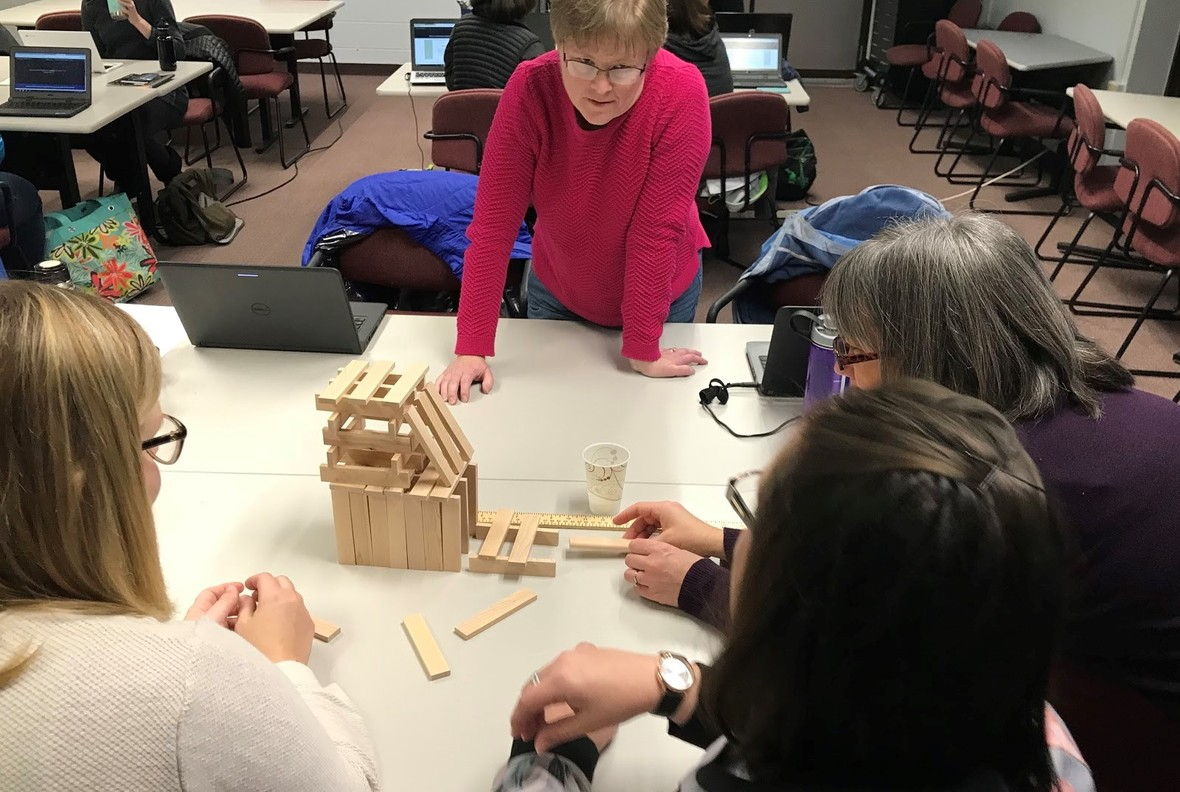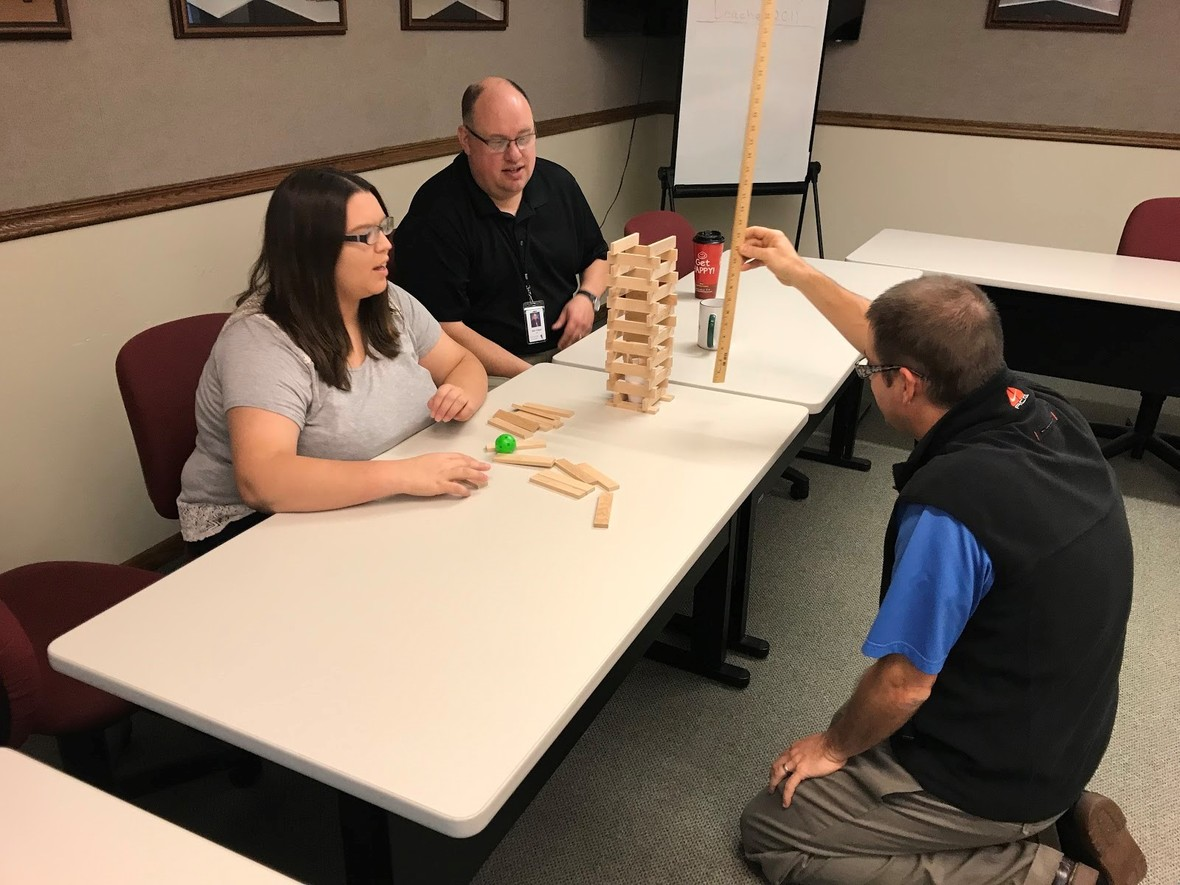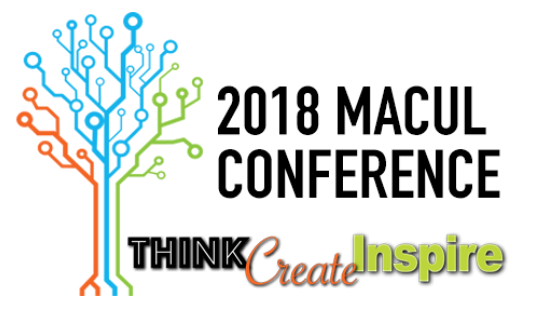Like   Tweet   +1
 /* styles */

## November 2017

 table div table+table+table+table div table{width:100%;padding:0}table div table+table+table+table div table img{width:96.23%;padding:0;float:none}table div table+table+table+table div table td{width:100%;padding:0 1.88% 18px}/* styles *//* styles */ Teachers representing the schools of Baraga, Gogebic, Houghton and Ontonagon counties met on October 27, 2017 and November 2, 2017 for the REMC1 Tech Academy. The Tech Academy is a program created by REMC1 and led by Educational Technologist, Steve Kass. The goal of Tech Academy is to foster collaboration among our local schools to discuss and share technology tools, resources, and integration ideas. Each school selects a teacher to represent them at the Academy and to bring back resources. Tech Academy will meet next on February 9 and questions can be directed to Steve Kass at steve@remc1.org(From top, moving clockwise: Melanie Harmala, Chassell Township Schools; Leona Blessing, Hancock Public Schools; Emily Maxson, L’Anse Area Schools; Jessica Klein, Lake Linden-Hubbell Public Schools.)(From left to right: Hilary Sundblad, Ontonagon Area School; Alan Tulppo, Ewen-Trout Creek School District; Bob Genisot, Gogebic-Ontonagon ISD)

 table div table+table+table+table+table+table+table+table+table div table{width:100%;padding:0}table div table+table+table+table+table+table+table+table+table div table img{width:96.23%;padding:0;float:none}table div table+table+table+table+table+table+table+table+table div table td{width:100%;padding:0 1.88% 18px}/* styles */## 2018 MACUL ConferenceThe MACUL Conference, Michigan's premier educational technology event, will be held March 7-9 at the DeVos Place in Grand Rapids, MI. Over 5,000 educators attend this conference annually and another year of record-level attendance is anticipated!

REMC1 recognizes the value of this conference and would like to assist our local schools in participating by providing the following accommodations:

Free Conference Registration & Bus Transportation to the conference for one person from your school district (Copper Country and Gogebic-Ontonagon ISD Schools only) Sign up here.

Discounted registration fee and bus fare for additional teachers to attend the conference from your district (We plan to reserve a charter bus again and subsidize the cost. We also coordinated with MACUL to receive a discount on registration through group pricing. Both of these items are dependent on reaching a minimum of 20 people from our region, though) Sign up here.

In order to receive the one complimentary registration and the discounted rates for additional teachers, REMC1 will need to register you with MACUL (DO NOT REGISTER ON MACUL's WEBSITE). Instead, please see our MACUL Registration & Bus Form. However, you will still need to book your hotel accommodation on your own and please visit MACUL's Conference Hotel Web page for reservations.

We are recommending the Holiday Inn and please reserve your hotel as soon as possible.
Additional information on the conference can be viewed at https://maculconference.org/ and questions can be directed to Steve Kass at steve@remc1.org

 table div table+table+table+table+table+table+table+table+table+table+table+table div table{width:100%;padding:0}table div table+table+table+table+table+table+table+table+table+table+table+table div table img{width:96.23%;padding:0;float:none}table div table+table+table+table+table+table+table+table+table+table+table+table div table td{width:100%;padding:0 1.88% 18px}/* styles */## Speak Up!Speak Up!

It’s time for students, parents, teachers, principals, librarians, technology leaders, superintendents and more to Speak Up! Share your thoughts and experiences on education issues like:
* Learning and teaching with mobile devices, digital content, gaming, videos, and virtual & augmented reality
* Internet access at home and in school
* Importance of math and best ways to learn math
* Digital citizenship
* And, much more!
Surveys take less than 20 minutes to complete and are completely anonymous. Join more than 500,000 people from more than 10,000 schools to be sure your voice is heard this year!

 table div table+table+table+table+table+table+table+table+table+table+table+table+table+table+table div table{width:100%;padding:0}table div table+table+table+table+table+table+table+table+table+table+table+table+table+table+table div table img{width:96.23%;padding:0;float:none}table div table+table+table+table+table+table+table+table+table+table+table+table+table+table+table div table td{width:100%;padding:0 1.88% 18px}/* styles */## PowerSchool "Unlimited Contacts"The latest release of PowerSchool (11.1) will feature “Unlimited Contacts”. This will completely change the way that contacts (parents and emergency contacts) are handled by PowerSchool. Instead of being limited to mother, father, emergency 1, emergency 2, emergency 3, there is now a choice of over ten types of contacts (including “Other”). Each student can have an unlimited amount of contacts; each contact can have an unlimited amount of e-mail addresses and phone numbers. This will also track when certain contacts are associated with the students (start date, end date).

Here are the features:

 Search for Contacts
 Web Access Account Integration
 Backward compatibility with core original contacts. (Mother, Father, Guardian, Emergency Contact 1-3 and all existing Access Accounts).
 Student Contacts page.
 Edit Relationship details
 Consolidate Contacts (To see feature: Click "Select by hand" from the Contact Search Results.)
 Import New Contacts in Data Import Manager (Import template will be posted soon.)
 View contact data on custom reports via new *Contact_Info Data Access Tag (Documentation will be posted soon.)
 New: Ability to Change Original Contact Type from Relationship Details
 New: Recovery Email for use with Web Access Accounts
 New: Guardian email integrated with contact emails
 New: Student Column displays on Contact search results
 New: More Countries seeded into the Country code set

There is currently a lab where you can log in and test-drive this new feature.

Jason Springel has mentioned unlimited contacts in an insider episode. Log in to PowerSource, click on the labs tab, and click the "unlimited contacts" link. Starts at 5:40.

 table div table+table+table+table+table+table+table+table+table+table+table+table+table+table+table+table+table+table div table{width:100%;padding:0}table div table+table+table+table+table+table+table+table+table+table+table+table+table+table+table+table+table+table div table img{width:96.23%;padding:0;float:none}table div table+table+table+table+table+table+table+table+table+table+table+table+table+table+table+table+table+table div table td{width:100%;padding:0 1.88% 18px}/* styles */## Teacher's CornerLots of good OER information at Open Educational Resources (OER): Resource Roundup

Nominate an outstanding tech leader for the 2018 #maculawards! Submit info here.

Bookshare is a way for teachers to assign books to students and has text to speech capabilities as well.

The Science of Opioid Addiction - Curriculum Resources - Aligned to national health and science standards, these resources introduce students to the science behind opioids and their impact on the brain and body.

Phelps Vs. Shark: The Battle for Ocean Supremacy - Explore the science behind this epic showdown between the greatest swimmer of all time, and the great white shark. This Shark Week program is now available in Streaming Plus, along with expertly crafted lesson plans!

New Discovery Educator Network Online Community - The DEN Online Community provides educators with more ways to learn from each other, all in one location, easily accessible through the navigation bar in Discovery Education. Collaborate with educators interested in similar things, share your strategies, ask questions, and find answers.

 table div table+table+table+table+table+table+table+table+table+table+table+table+table+table+table+table+table+table+table+table+table div table{width:100%;padding:0}table div table+table+table+table+table+table+table+table+table+table+table+table+table+table+table+table+table+table+table+table+table div table img{width:96.23%;padding:0;float:none}table div table+table+table+table+table+table+table+table+table+table+table+table+table+table+table+table+table+table+table+table+table div table td{width:100%;padding:0 1.88% 18px}/* styles */## ResourcesEduPaths is a free resource from TRIG created to centralize PD around the state. Find resources and get FREE SCECH hours!

Current REMC Connect (REMC Association of Michigan Newsletter)

Check out Discovery Streaming updates and enhancing instruction with streaming resources.

MeL is a great place for Michigan Teachers to find classroom resources. They have just recently launched MeL Teachers which offers eight centers that will help with site navigation and make important information easier to access.

 table div table+table+table+table+table+table+table+table+table+table+table+table+table+table+table+table+table+table+table+table+table+table+table+table div table{width:100%;padding:0}table div table+table+table+table+table+table+table+table+table+table+table+table+table+table+table+table+table+table+table+table+table+table+table+table div table img{width:96.23%;padding:0;float:none}table div table+table+table+table+table+table+table+table+table+table+table+table+table+table+table+table+table+table+table+table+table+table+table+table div table td{width:100%;padding:0 1.88% 18px}/* styles */## Connection Cornertable div table+table+table+table+table+table+table+table+table+table+table+table+table+table+table+table+table+table+table+table+table+table+table+table+table+table+table div table{width:100%;padding:0}table div table+table+table+table+table+table+table+table+table+table+table+table+table+table+table+table+table+table+table+table+table+table+table+table+table+table+table div table img{width:96.23%;padding:0;float:none}table div table+table+table+table+table+table+table+table+table+table+table+table+table+table+table+table+table+table+table+table+table+table+table+table+table+table+table div table td{width:100%;padding:0 1.88% 18px}/* styles */table div table+table+table+table+table+table+table+table+table+table+table+table+table+table+table+table+table+table+table+table+table+table+table+table+table+table+table+table+table div table{width:100%;padding:0}table div table+table+table+table+table+table+table+table+table+table+table+table+table+table+table+table+table+table+table+table+table+table+table+table+table+table+table+table+table div table img{width:96.23%;padding:0;float:none}table div table+table+table+table+table+table+table+table+table+table+table+table+table+table+table+table+table+table+table+table+table+table+table+table+table+table+table+table+table div table td{width:100%;padding:0 1.88% 18px}/* styles *//* styles */ The REMC office will be closed the following days during the holiday season: Thursday, November 23, 2017 Friday, November 24, 2017 Monday, December 25, 2017 Tuesday, December 26, 2017 Friday, December 29, 2017 Monday, January 1, 2018
 table div table+table+table+table+table+table+table+table+table+table+table+table+table+table+table+table+table+table+table+table+table+table+table+table+table+table+table+table+table+table+table div table{width:100%;padding:0}table div table+table+table+table+table+table+table+table+table+table+table+table+table+table+table+table+table+table+table+table+table+table+table+table+table+table+table+table+table+table+table div table img{width:96.23%;padding:0;float:none}table div table+table+table+table+table+table+table+table+table+table+table+table+table+table+table+table+table+table+table+table+table+table+table+table+table+table+table+table+table+table+table div table td{width:100%;padding:0 1.88% 18px}/* styles */table div table+table+table+table+table+table+table+table+table+table+table+table+table+table+table+table+table+table+table+table+table+table+table+table+table+table+table+table+table+table+table+table+table div table{width:100%;padding:0}table div table+table+table+table+table+table+table+table+table+table+table+table+table+table+table+table+table+table+table+table+table+table+table+table+table+table+table+table+table+table+table+table+table div table img{width:96.23%;padding:0;float:none}table div table+table+table+table+table+table+table+table+table+table+table+table+table+table+table+table+table+table+table+table+table+table+table+table+table+table+table+table+table+table+table+table+table div table td{width:100%;padding:0 1.88% 18px}/* styles */table div table+table+table+table+table+table+table+table+table+table+table+table+table+table+table+table+table+table+table+table+table+table+table+table+table+table+table+table+table+table+table+table+table+table+table div table{width:100%;padding:0}table div table+table+table+table+table+table+table+table+table+table+table+table+table+table+table+table+table+table+table+table+table+table+table+table+table+table+table+table+table+table+table+table+table+table+table div table img{width:96.23%;padding:0;float:none}table div table+table+table+table+table+table+table+table+table+table+table+table+table+table+table+table+table+table+table+table+table+table+table+table+table+table+table+table+table+table+table+table+table+table+table div table td{width:100%;padding:0 1.88% 18px}/* styles */